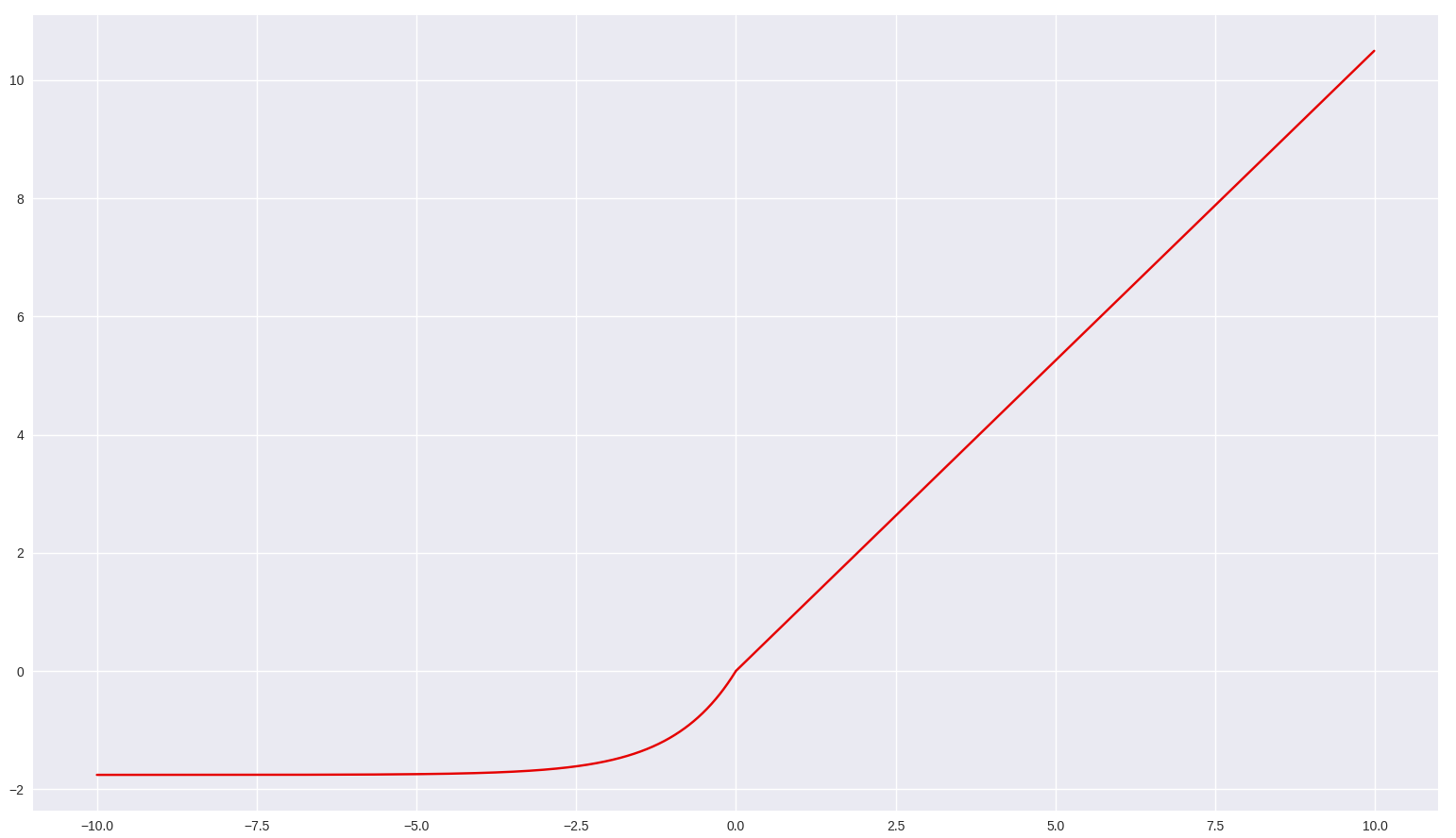Missed TensorFlow Dev Summit? Check out the video playlist.

# tf.keras.activations.selu

Scaled Exponential Linear Unit (SELU).

``````tf.keras.activations.selu(
x
)
``````

The Scaled Exponential Linear Unit (SELU) activation function is: `scale * x` if `x > 0` and `scale * alpha * (exp(x) - 1)` if `x < 0` where `alpha` and `scale` are pre-defined constants (`alpha = 1.67326324` and `scale = 1.05070098`). The SELU activation function multiplies `scale` > 1 with the `[elu](https://www.tensorflow.org/versions/r2.0/api_docs/python/tf/keras/activations/elu)` (Exponential Linear Unit (ELU)) to ensure a slope larger than one for positive net inputs.

The values of `alpha` and `scale` are chosen so that the mean and variance of the inputs are preserved between two consecutive layers as long as the weights are initialized correctly (see `lecun_normal` initialization) and the number of inputs is "large enough" (see references for more information).(Courtesy: Blog on Towards DataScience at towardsdatascience.com/selu-make-fnns-great-again-snn-8d61526802a9)

#### Example Usage:

````n_classes = 10  #10_class problem `
`from tensorflow.python.keras.layers import Dense `
`model = tf.keras.Sequential() `
`model.add(Dense(64, kernel_initializer='lecun_normal', `
`                activation='selu', input_shape=(28, 28, 1))) `
`model.add(Dense(32, kernel_initializer='lecun_normal', `
`                activation='selu')) `
`model.add(Dense(16, kernel_initializer='lecun_normal', `
`                activation='selu')) `
`model.add(Dense(n_classes, activation='softmax')) `
```

#### Arguments:

• `x`: A tensor or variable to compute the activation function for.

#### Returns:

The scaled exponential unit activation: `scale * elu(x, alpha)`.

# Note

``````- To be used together with the initialization "[lecun_normal]
(https://www.tensorflow.org/api_docs/python/tf/keras/initializers/lecun_normal)".
- To be used together with the dropout variant "[AlphaDropout]